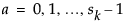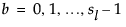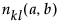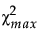Suppose that a design has n runs and p factors corresponding to the columns of the design matrix.
 • Denote the levels of factors k and l byand, respectively.
 • Denote the number of times that the combination of levels (a,b) appears in columns k and l by.
A measure of the lack of orthogonality evidenced by columns k and l is given by the following expression:
The maximum possible value of χ2, denoted, is obtained. The chi-square efficiency of a design is defined as follows:
Chi-square efficiency indicates how close χ2 is to zero, relative to a design in which pairs of levels show extreme lack of balance.

Help created on 9/19/2017